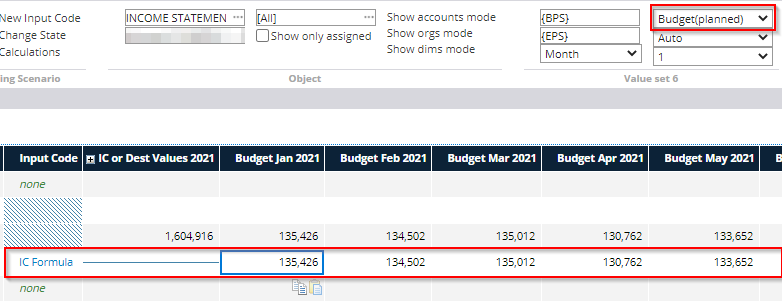This article discusses the features of the Formula input code that can used to create calculation formulas using a separate formula editor.

Input Code Properties -tab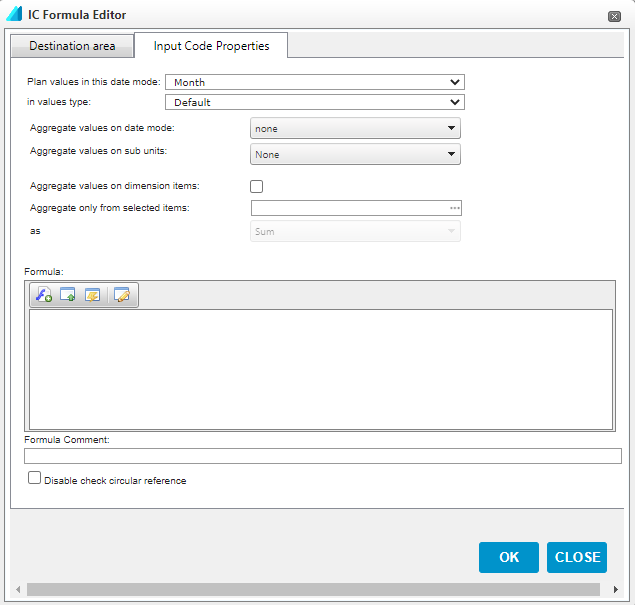Plan values in this date mode: This setting determines the accuracy level at which the calculation is performed. With monthly accuracy, a separate percentage is determined for each month, while, for example, with annual accuracy, only one percentage value is determined for the entire year.

In values type: Specifies the type of calculated values stored in the system. In most cases, you can use the default setting, in which case the type of values calculated depends on the settings made on the chart of accounts.

Aggregate values on date mode/sub units: If multiplication is used in the formula, these settings can be used to determine how the calculated result for individual months or cost centers is added to the higher levels. It is usually not necessary to make changes to this setting.

Disable check circular reference: Allows the use of circular references in the input code, i.e. references that depend on the results of the same formula.

Formula Editor: At the bottom of the dialog box, the calculation formula for the input code can be defined. The formula editor supports the following characters: +, -, *, /, =, | (“Or”), & (“and”), (). A dot is used as a decimal separator in the editor.

In addition to writing a formula, various functions or references to specific accounts or report rows can be added to the editor.

Functions: Functions are used in more complex calculations making it possible to include conditional expressions in the formula. A function is added from the first formula editor selection button.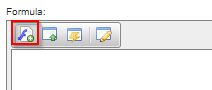This opens a dialog box. The function is selected from the drop-down menu.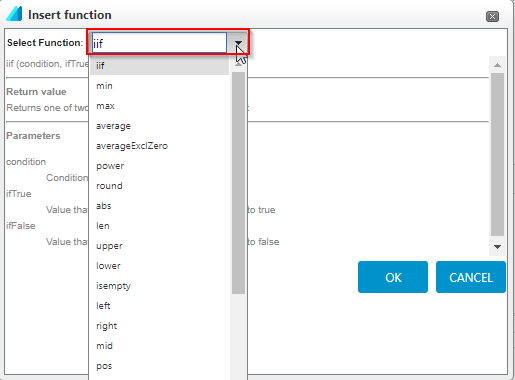References: References can refer to a specific account, report line, or account type in a formula. A reference is added from the second formula editor selection button.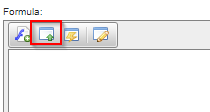This opens a dialog box that specifies the reference parameters.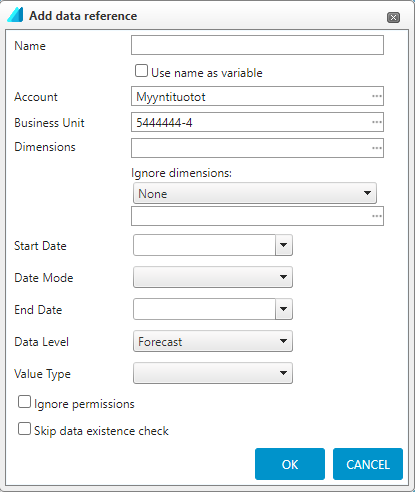Name: Optional information. This field can be left blank, in which case the system will name the reference automatically based on the parameters entered.

Account / Report Line / Account Type: Required information. Choose from the drop-down menu whether you want to refer to an account, a report line item, or an account type. The actual information is selected from the field.

Business Unit: Optional. Selecting an organization level in this field will force you to always retrieve a value from a specific item, regardless of the level at which the input code was originally created.

Dimensions: Optional information. Works in the same way as the Business Unit.

Ignore dimensions: Optional. This setting is used when you want to ignore a dimension object in the formula calculation. Options for skipping dimensions are: All, Only Selected , and Except Selected.

Start Date and End Date: Optional. You can enter a time interval in these fields if you want to force the reference to a specific time period.

Date Mode: Optional. With this setting, the reference can be calculated from a specific time period, eg the 'Year' selection calculates the value of the whole year for the reference (together with the From and To fields).

Data level: Optional. Indicates from which data level the reference values are retrieved.

Value type: Optional. This determines the type of values in which the result of the reference is given, eg for balance sheet references, the value type can be set to 'Closing balance'.

The only required information for these parameters is Account / Report Line / Account Type. Other options can be used when needed. If the parameter is left blank, the data for the level at which the input code was created is used to calculate the formula. For example, if an input code is created for the budget entry template and the Administration Cost Center, the formula automatically uses this Organization Level and Data Level, even if they are not selected for the reference parameters above.

Example: External services calculated by a formula

In the example below, a formula calculates forecast values for External Services 17% of the difference between Revenue and Purchases during the financial year.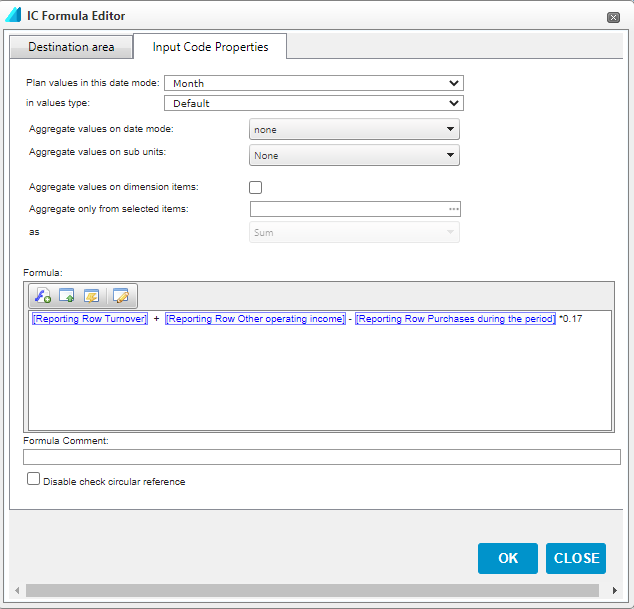References do not require anything other than an account, report row, or account group. There is no need to choose the business unit, data level, or other parameters as the input code automatically calculates the formula from the level at which it was created.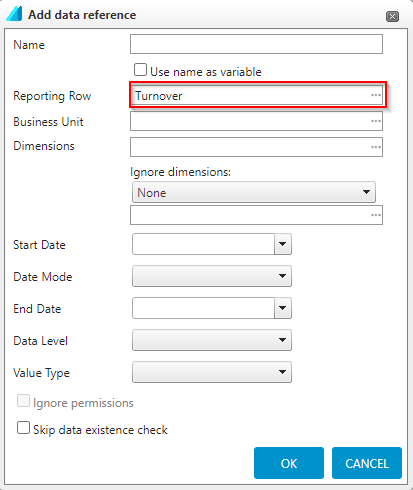Destination area -tab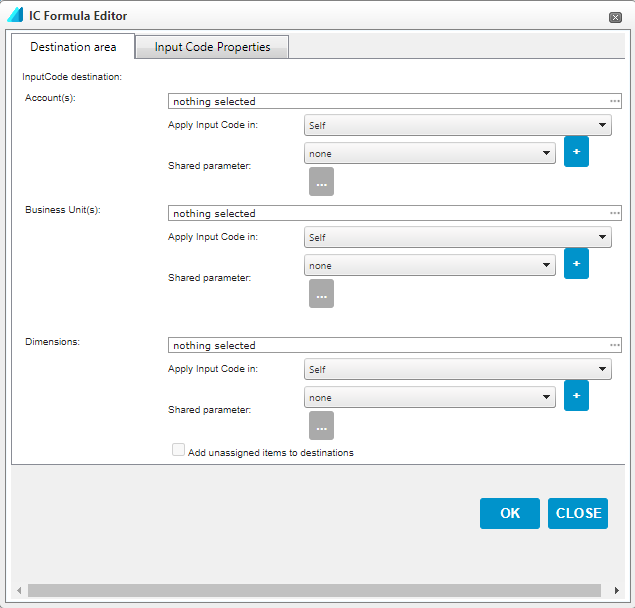Account(s): Select the accounts / account groups for which the input code is used

Business Unit(s): The organizational levels at which the input code is used

Dimensions(s): Dimensions or dimension objects for which the input code is used. By selecting 'Add unassigned items to destinations', the calculation is also performed for values that are not dimensioned

For all of the above parameters, there are two choices to determine how extensively the calculation is performed:

Apply Input Code in: Specifies whether the input code is used only at the selected level, its cost centers, or parallel levels

Shared parameter: A common parameter that contains user-defined organizational levels or accounts  can be selected in this field. This function can be used if you want to add several different input codes to the same set of accounts or organizations, without defining them in each input code separately. A new common parameter is created from the + sign.

Once all the above parameters have been defined, select 'OK' to create the input code.

Running the calculation and viewing the results

The input code (and its formula) is calculated by selecting Run from the bar of the input template.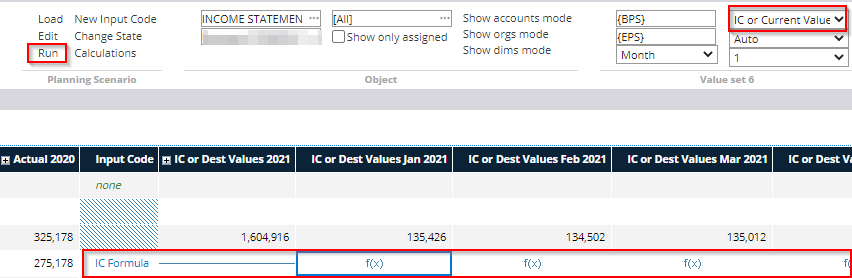The values calculated with the input code can be viewed by selecting Budget or Planned as the display format for the input template, as shown, depending on whether the input template is Budget or Forecast.